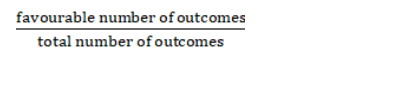# From a well-shuffled pack of cards, a card is drawn at random.

Question:

From a well-shuffled pack of cards, a card is drawn at random. Find the probability of its being either a queen or a heart.

Solution:

let A denote the event that the card drawn is queen and B denote the event that card drawn is the heart.

In a pack of 52 cards, there are 4 queen cards and 13 heart cards

Given: $P(A)=\frac{4}{52}, P(B)=\frac{13}{52}$

To find : Probability that card drawn is either a queen or heart = P(A or B)

The formula used : Probability =$P(A$ or $B)=P(A)+P(B)-P(A$ and $B)$

$P(A)=\frac{4}{52}$ (as favourable number of outcomes $=4$ and total number of outcomes $\left.=52\right)$

$P(B)=\frac{13}{52}$ (as favourable number of outcomes $=13$ and total number of outcomes $\left.=52\right)$

Probability that card drawn is both queen and heart $=P(A$ and $B)=1$

(as there is one card which is both queen and heart i.e queen of hearts)

$P(A$ or $B)=\frac{4}{52}+\frac{13}{52}-1$

$P(A$ or $B)=\frac{4+13-1}{52}=\frac{16}{52}=\frac{4}{13}$

$P(A$ or $B)=\frac{4}{13}$

Probability of a card drawn is either a queen or heart $=\mathrm{P}(\mathrm{A}$ or $\mathrm{B})=\frac{4}{13}$